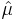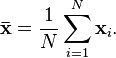# Method of Moments Definition and Example

Statistics Definitions > Method of Moments

## What is Method of Moments?

The method of moments is a way to estimate population parameters, like the population mean or the population standard deviation. The basic idea is that you take known facts about the population, and extend those ideas to a sample. For example, it’s a fact that within a population:
Expected value E(x) = μ
For a sample, the estimatoris just the sample mean, . The formula for the sample mean is:This is the first moment condition.

The second moment condition involves the variance. The population variance is Var(x) = σ2, so we just need to use the method of moments to estimate the variance in the sample. Here’s how the formula is derived:

The above method is probably the most widely used method of moments. There is another method, which uses sample moments about the mean instead of sample moments about the origin.